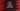# Dart number datatype : integers and double### Dart Datatype: Numbers (integers and double):

While creating variables, we need to define its type. For example, if it is string variable or a numerical variable. Dart also provides different datatypes. We will learn about each datatype one by one. This tutorial will explain you about Number datatype in Dart. The first thing that comes to our mind is integers if we talk about numbers. In dart, we have two types of number variables: integers and doubles.

### Integers :

The maximum size of an integer is 64 bit. That means, it is in the range -2^63 to 2^63 - 1. Some examples of integer are 2,3,4,50,432,0xEDBEAFAA etc.

### Doubles :

Doubles are IEEE 754 standard floating point numbers. The size of the double is 64 bit. Integers don’t contain any decimal point, but double contains a decimal point. Some examples of double are 12.3,4.2,133.23 etc.

### Doing numerical operations on a number :

We can perform basic numerical operations like addition, subtraction, multiplication and division on two dart numbers. Let me show you with an example :

``````main(){
int two = 2;
int four = 4;

double fiveDouble = 5.1;
double sixDouble = 6.1;

int mul = two * four;
double add = fiveDouble + sixDouble;
double sub = sixDouble - fiveDouble;
double div = four/two;

print("Multiplying two with four = \$mul ");
print("Subtract fiveDouble from sixDouble = \$sub");
print("Divide four by two = \$div");

}
``````

It will print :

``````Multiplying two with four = 8
Adding fiveDouble with sixDouble = 11.2
Subtract fiveDouble from sixDouble = 1.0
Divide four by two = 2.0
``````

The last number 2.0 is a double, we have done division on two integers and the result is double.

### Parsing a string to integer or double in Dart :

In dart, you can convert any string to a number, i.e. to an integer or double using parse() method. Let’s try to understand it with an example :

``````main(){
int two = int.parse("2");
double threeDouble = double.parse("3.5");

print("value of two is \$two");
print("value of three double is \$threeDouble");
}
``````

Output :

``````value of two is 2
value of three double is 3.5
``````

If you will try to parse one string holding one integer value to double, it will produce one double :

``````double threeDouble = double.parse("3");
``````

threeDouble is holding 3.0. But, the opposite is not true,i.e. if we have one double number as a string and if we try to parse it as an integer, it will throw one error. Following example will throw one FormatException.

``````int two = int.parse("2.3");
``````

Similarly, if you try to parse any other string, it will throw FormatException.

### Few important methods and properties of integer :

1. isEven: It will check if the integer is even or not. If yes, it will return true, false otherwise.
2. isOdd: Returns true if it is odd, else false.
3. sign: Returns the sign of the integer. 0 for zero, -1 for negative and +1 for positive.
4. isNaN: Returns true if it is a double Not-a-Number value, else false.
5. isNegative : true if the number is negative, else false.
6. abs() method: Returns the absolute value of the integer.
7. gcd(int other): Returns the gcd of this integer and other integers.
8. toString(): Returns the string representation of the integer.
9. compareTo(int other): Compare the current integer with other integers.
10. remainder(int other): Returns the remainder by dividing this integer by other.

These are few sample methods and properties of dart integer. You can check all properties and methods for integer using this link and similarly for double use this.